• Home
• JEE Main Paper 2 Apt...

# JEE Main Paper 2 Aptitude Questions 2024

Author : Palak Khanna

Updated On : July 11, 2023

SHARE

Summary: This article has provided you with a list of most expected JEE Main Paper 2 Aptitude Questions with solutions. Remember, the only way to score good marks in the aptitude section is by solving these questions regularly.

The first JEE Main Paper 2 exam session is scheduled in the year 2023 and the second session will precede the first session.

Most of you might be worried about enhancing your speed and accuracy to solve aptitude questions, as we all know how well prepared you need to be for this entrance exam. Remember, practice is the only key to scoring good marks in the aptitude section.

To help you get an idea about the type of questions asked and their difficulty level, this post comprises of most expected JEE Main Paper 2 Aptitude Questions with solutions.

So, what are you looking for? Try to practice these questions regularly and perform well in the upcoming exam.

"Most of you might be worried about enhancing your speed and accuracy to solve aptitude questions, as we all know how well prepared you need to be for this entrance exam. Remember, practice is the only key to scoring good marks in the aptitude section."

## JEE Main Paper 2 Aptitude Questions 2023

As per the JEE Main Paper 2 Exam Pattern,  a total of 50 questions are asked under the aptitude section. For every correct answer, you will be awarded four marks.

Also, there is a negative marking in the aptitude section. For every wrong answer, you will lose a -1 mark.

Managing time is an essential factor while solving aptitude questions. Each question may consume different timings; hence you must ensure not to spend too much time on a question when you cannot find the answer.

"Managing time is an essential factor while solving aptitude questions. Each question may consume different timings; hence you must ensure not to spend too much time on a question when you cannot find the answer."

## Important Quantitative Aptitude Questions for JEE Main Paper 2

In the aptitude section, you will be tested mainly on objects, texture related to architecture, building environment, visualizing different sides of three-dimensional objects, analytical reasoning & mental ability, design and drawing of geometrical or abstract shapes and patterns in pencil, etc.

Here are a few sample aptitude questions curated from the previous year's JEE Main Paper 2 question papers.

Try solving them to analyze your preparation levels for the upcoming exam.

1. From 6 different novels and 3 different dictionaries, 4 novels, and 1 dictionary are to be selected and arranged in a row on a shelf so that the dictionary is always in the middle. The number of such arrangements is:

A) at least 750 but less than 1000

B) at least 1000

C) less than 500

D) at least 500 but less than 750

2. A straight line through a fixed point (2, 3) intersects the coordinate axes at distinct points P and Q. If O is the origin and the rectangle OPRQ is completed, then the locus of R is:

A) 3x + 2y = 6xy

B) 3x + 2y = 6

C) 2x + 3y = xy

D) 3x + 2y = xy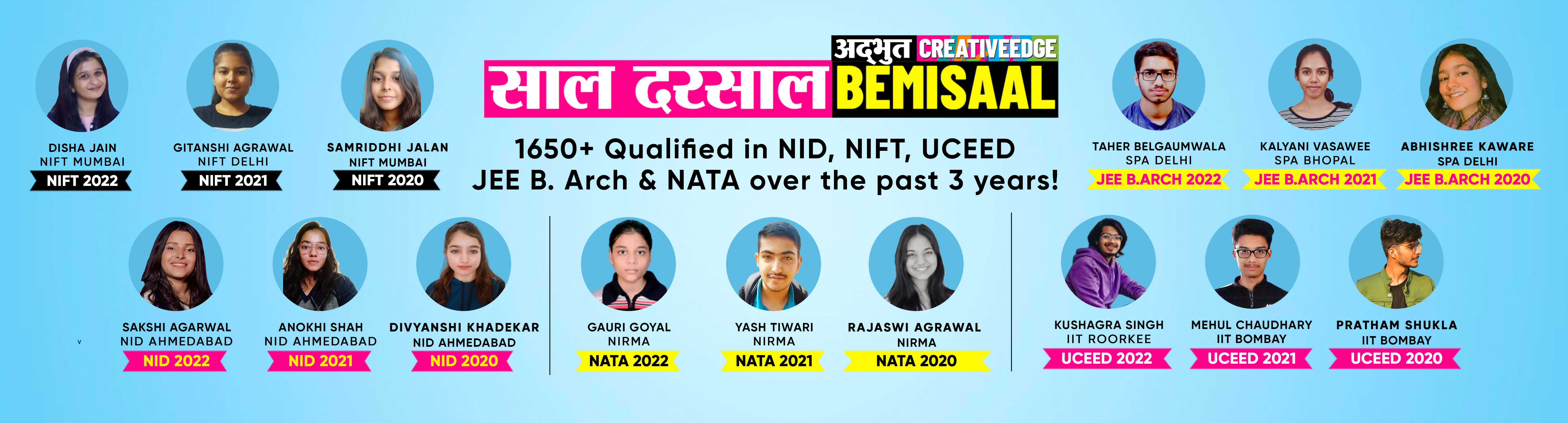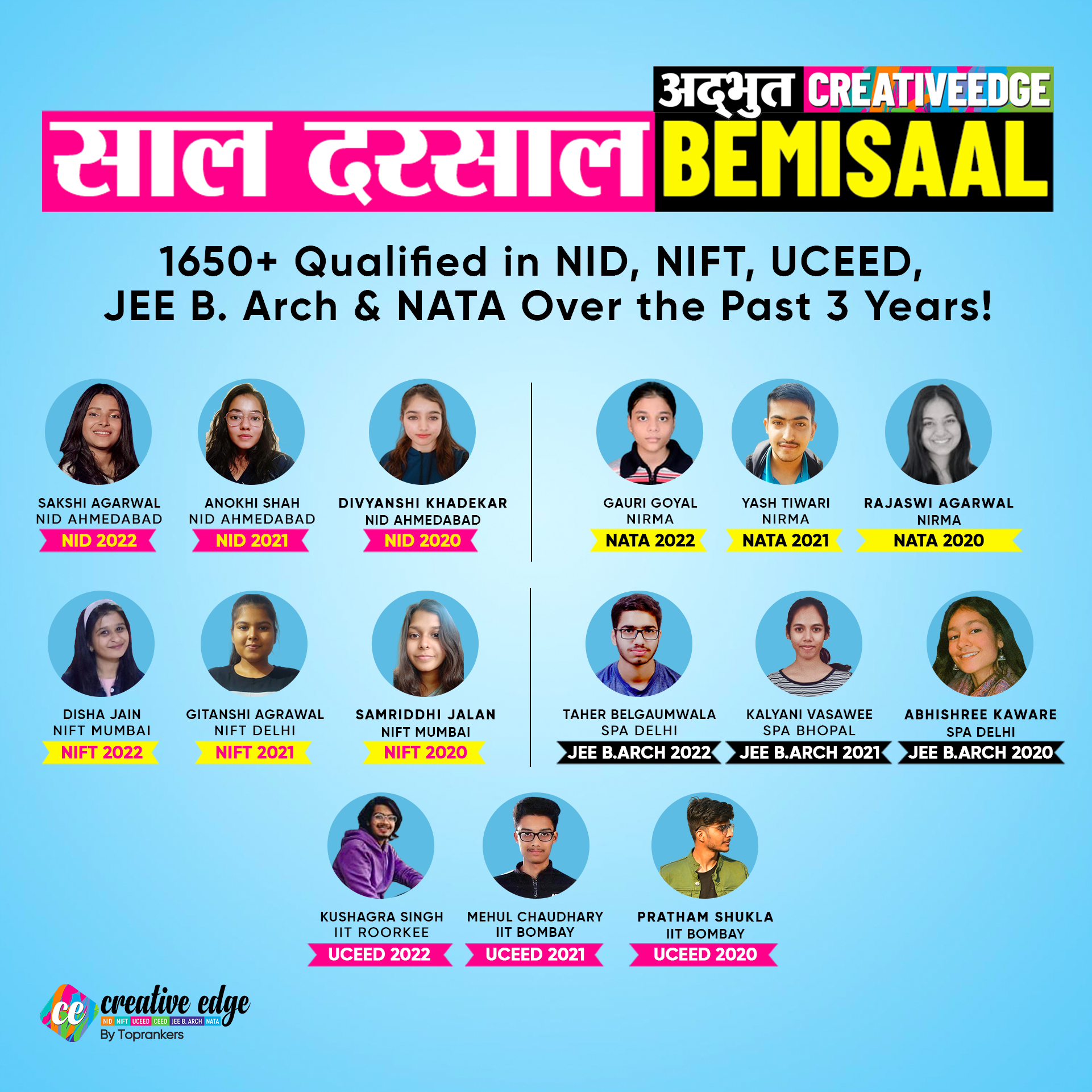3. Tangent and normal are drawn at P (16, 16) on the parabola y square = 16x, which intersect the axis of the parabola at A and B, respectively. If C is the center of the circle through the points P, A, and B and angle CPB = 𝜃, then a value of tan 𝜃 is.

4. A certain type of bacteria reproduces itself at the rate of 10% every 5 minutes. In how many minutes will the number double itself?

1) 20 minutes

2) 30 minutes

3) 40 minutes

4) None of these

5. If the points (– 1, 2, – 3), (4, a, 1), and (b, 8, 5) are collinear then the values of a and b respectively will be

1) 5, 5

2) 9, 5

3) 5, 9

4) – 5, 9

Read more: Important topics for JEE Main Paper 2 Exam

6. If on average 1 vessel out of 10 is wrecked, the probability that out of 5 vessels expected to arrive, 4 at least will arrive safely is.

1) 45927/50000

2) 4592/50000

3) 8627/5000

4) None of these

7. A family has 5 children. Assuming that the probability of a girl on each birth was ½ and five births are independent. The probability that the family has at least one girl given they have at least one boy is.

1) 31/32

2) 30/31

3) 15/16

4) 15/31

8. A and B throw alternately with a pair of dice, A wins if he throws 6 before B throws 7 and B wins if he throws 7 before A throws 6. If A begins then his chance of winning is.

9. In an increasing geometric series, the sum of the first and the sixth term is 66 and the product of the second and the fifth terms are 128. Then the sum of the first 6 terms of this series is:

1) 127

2) 129

3) 126

4) 128

10. Let A(1,3) and C(5,1) be two opposite vertices of a rectangle. The other two vertices B(a,b) and D(c,d) lie on the line y=2x+k for some k. Then the value of (a+b)*(c+d) is:

1) 8

2) 16

3) 24

4) 32

## JEE Main Paper 2 General Aptitude Questions

Along with proper planned preparation for JEE Main Paper 2, you need to practice more questions to secure good marks.

1. A small lift for carrying a small load only is known as:

A) A deaf bearer

B) A dumb waiter

C) A jockey boy

D) A push upper

2. Which of the following is the most famous temple in the Khajuraho Group of Temples?

A) Shiva Temple

C) Ganesh Temple

D) Krishna Temple

3. Where is Mehrangarh fort located?

A) Jodhpur

B) Udaipur

C) Jaisalmer

D) Jaipur

4. Where is “Hathi Park” located?

A) Bengaluru

B) Kochi

C) Chennai

D) Lucknow

5. Which variety of Glass is heat resistant?

A) Flint Glass

B) Battle Glass

C) Sheet Glass

D) Pyrex Glass

6. Qutub Minar in Delhi was built by?

A) Shahjahan

B) Iltutmuch

C) Qutubuddin Aibak

D) Akbar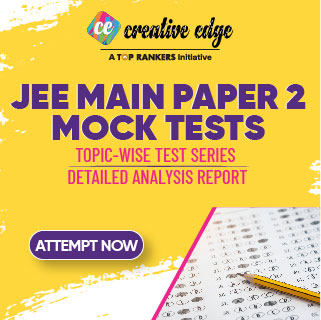7. Identify the correct elevation of the figure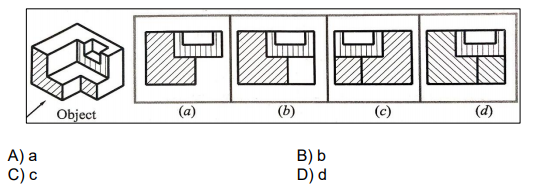8. Select a figure from amongst the Answer Figures which will continue the same series as established by the five Problem Figures.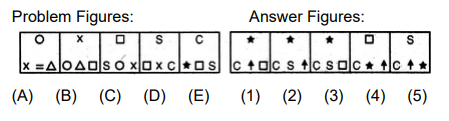9. Find out the alternative figure which contains figure (X) as its part.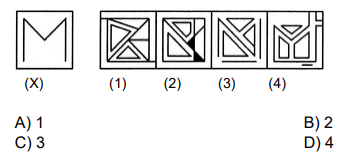10. Select a figure from amongst the Answer Figures which will continue the same series as established by the five Problem Figures.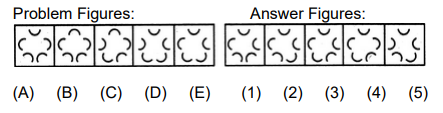What is aptitude test in JEE Main Paper 2?How many questions are asked in JEE Main Paper 2 Aptitude section?What is the mode of JEE Main Paper 2 aptitude test?What is the JEE Main paper 2 aptitude test 2024 syllabus?Is the aptitude test syllabus the same for B. Arch and B. Planning programs?Is there negative marking in the JEE Main paper 2 exam 2024?What is the salary of B. Arch?